•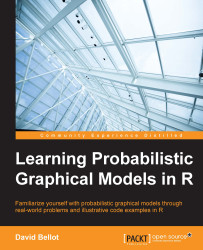#### Learning Probabilistic Graphical Models in R#### Overview of this book

Probabilistic graphical models (PGM, also known as graphical models) are a marriage between probability theory and graph theory. Generally, PGMs use a graph-based representation. Two branches of graphical representations of distributions are commonly used, namely Bayesian networks and Markov networks. R has many packages to implement graphical models. We’ll start by showing you how to transform a classical statistical model into a modern PGM and then look at how to do exact inference in graphical models. Proceeding, we’ll introduce you to many modern R packages that will help you to perform inference on the models. We will then run a Bayesian linear regression and you’ll see the advantage of going probabilistic when you want to do prediction. Next, you’ll master using R packages and implementing its techniques. Finally, you’ll be presented with machine learning applications that have a direct impact in many fields. Here, we’ll cover clustering and the discovery of hidden information in big data, as well as two important methods, PCA and ICA, to reduce the size of big problems.
Learning Probabilistic Graphical Models in RCreditswww.PacktPub.comPrefaceFree Chapter
Probabilistic ReasoningExact InferenceLearning ParametersBayesian Modeling – Basic ModelsApproximate InferenceBayesian Modeling – Linear ModelsProbabilistic Mixture ModelsAppendixIndex## Importance sampling

Importance sampling is an improvement on rejection sampling. Again the assumptions are the same and we will use a proposal distribution q(x). We also assume that we can compute the value of the density of probability p(x). But we are unable to draw a sample from it because it is, again, too complex.

Importance sampling is based on the following reasoning, where we need to evaluate the expectation of a function f(x) with respect to the distribution p(x):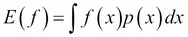At this stage, we simply introduce the distribution q(x) in the previous expression: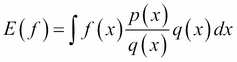And, as before, we approximate it with a finite sum: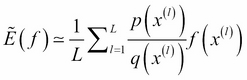The ratio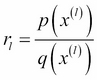is called importance weight and it is the bias introduced by sampling q(x) when in fact we wanted to sample from p(x). In this case, the algorithm is very simple because all the samples are used. Again, importance sampling is efficient if the proposal distribution is close enough to the original distribution. If the function f(x) varies a lot, we might end up in a situation where...